Documentation

# scatter3

3-D scatter plot

## Syntax

``scatter3(X,Y,Z)``
``scatter3(X,Y,Z,S)``
``scatter3(X,Y,Z,S,C)``
``scatter3(___,'filled')``
``scatter3(___,markertype)``
``scatter3(___,Name,Value)``
``scatter3(ax,___)``
``h = scatter3(___)``

## Description

example

````scatter3(X,Y,Z)` displays circles at the locations specified by the vectors `X`, `Y`, and `Z`. ```

example

````scatter3(X,Y,Z,S)` draws each circle with the size specified by `S`. To plot each circle with equal size, specify `S` as a scalar. To plot each circle with a specific size, specify `S` as a vector.```

example

````scatter3(X,Y,Z,S,C)` draws each circle with the color specified by `C`. If `C` is a RGB triplet or character vector or string containing a color name, then all circles are plotted with the specified color. If `C` is a three column matrix with the number of rows in `C` equal to the length of `X`, `Y`, and `Z`, then each row of `C` specifies an RGB color value for the corresponding circle. If `C` is a vector with length equal to the length of `X`, `Y`, and `Z`, then the values in `C` are linearly mapped to the colors in the current colormap. ```

example

````scatter3(___,'filled')` fills in the circles, using any of the input argument combinations in the previous syntaxes.```

example

````scatter3(___,markertype)` specifies the marker type.```

example

````scatter3(___,Name,Value)` modifies the scatter chart using one or more name-value pair arguments. ```

example

````scatter3(ax,___)` plots into the axes specified by `ax` instead of into the current axes (`gca`). The `ax` option can precede any of the input argument combinations in the previous syntaxes.```

example

````h = scatter3(___)` returns the `Scatter` object. Use `h` to modify properties of the scatter chart after it is created.```

## Examples

collapse all

Create a 3-D scatter plot. Use `sphere` to define vectors `x`, `y`, and `z`.

```figure [X,Y,Z] = sphere(16); x = [0.5*X(:); 0.75*X(:); X(:)]; y = [0.5*Y(:); 0.75*Y(:); Y(:)]; z = [0.5*Z(:); 0.75*Z(:); Z(:)]; scatter3(x,y,z)```Use `sphere` to define vectors `x`, `y`, and `z`.

```[X,Y,Z] = sphere(16); x = [0.5*X(:); 0.75*X(:); X(:)]; y = [0.5*Y(:); 0.75*Y(:); Y(:)]; z = [0.5*Z(:); 0.75*Z(:); Z(:)];```

Define vector `s` to specify the marker sizes.

```S = repmat([100,50,5],numel(X),1); s = S(:);```

Create a 3-D scatter plot and use `view` to change the angle of the axes in the figure.

```figure scatter3(x,y,z,s) view(40,35)```Corresponding entries in `x`, `y`, `z`, and `s` determine the location and size of each marker.

Use `sphere` to define vectors `x`, `y`, and `z`.

```[X,Y,Z] = sphere(16); x = [0.5*X(:); 0.75*X(:); X(:)]; y = [0.5*Y(:); 0.75*Y(:); Y(:)]; z = [0.5*Z(:); 0.75*Z(:); Z(:)];```

Define vectors `s` and `c` to specify the size and color of each marker.

```S = repmat([50,25,10],numel(X),1); C = repmat([1,2,3],numel(X),1); s = S(:); c = C(:);```

Create a 3-D scatter plot and use `view` to change the angle of the axes in the figure.

```figure scatter3(x,y,z,s,c) view(40,35)```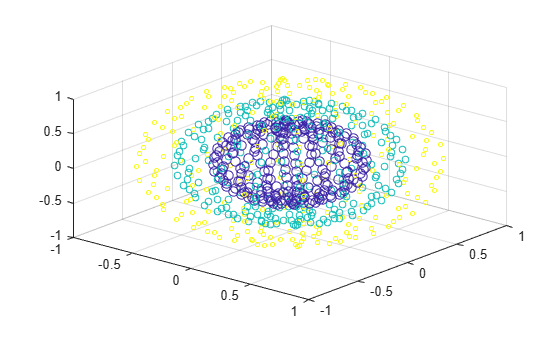Corresponding entries in `x`, `y`, `z`, and `c` determine the location and color of each marker.

Create vectors `x` and `y` as cosine and sine values with random noise.

```z = linspace(0,4*pi,250); x = 2*cos(z) + rand(1,250); y = 2*sin(z) + rand(1,250);```

Create a 3-D scatter plot and fill in the markers. Use `view` to change the angle of the axes in the figure.

```scatter3(x,y,z,'filled') view(-30,10)```Initialize the random-number generator to make the output of `rand` repeatable. Define vectors `x` and `y` as cosine and sine values with random noise.

```rng default z = linspace(0,4*pi,250); x = 2*cos(z) + rand(1,250); y = 2*sin(z) + rand(1,250);```

Create a 3-D scatter plot and set the marker type. Use `view` to change the angle of the axes in the figure.

```figure scatter3(x,y,z,'*') view(-30,10)```Initialize the random-number generator to make the output of `rand` repeatable. Define vectors `x` and `y` as cosine and sine values with random noise.

```rng default z = linspace(0,4*pi,250); x = 2*cos(z) + rand(1,250); y = 2*sin(z) + rand(1,250);```

Create a 3-D scatter plot and set the marker edge color and the marker face color. Use `view` to change the angle of the axes in the figure.

```figure scatter3(x,y,z,... 'MarkerEdgeColor','k',... 'MarkerFaceColor',[0 .75 .75]) view(-30,10)```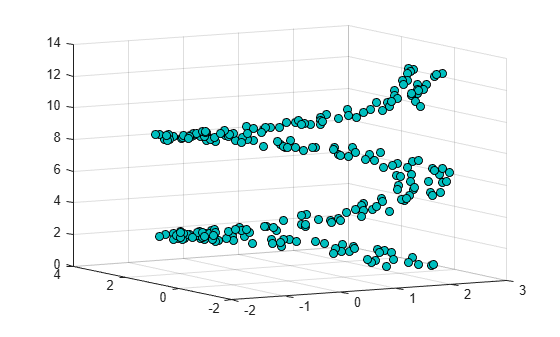Starting in R2019b, you can display a tiling of plots using the `tiledlayout` and `nexttile` functions.

Load the `seamount` data set to get vectors `x`, `y`, and `z`. Call the `tiledlayout` function to create a 2-by-1 tiled chart layout. Call the `nexttile` function to create the axes objects `ax1` and `ax2`. Then create separate scatter plots in the axes by specifying the axes object as the first argument to `scatter3`.

```load seamount tiledlayout(2,1) ax1 = nexttile; ax2 = nexttile; scatter3(ax1,x,y,z,'MarkerFaceColor',[0 .75 .75]) scatter3(ax2,x,y,z,'*')```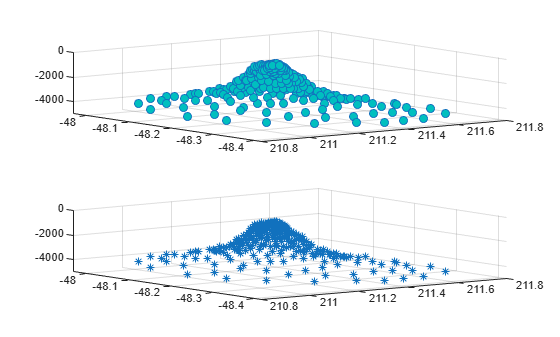Use the `sphere` function to create vectors `x`, `y`, and `z`.

```[X,Y,Z] = sphere(16); x = [0.5*X(:); 0.75*X(:); X(:)]; y = [0.5*Y(:); 0.75*Y(:); Y(:)]; z = [0.5*Z(:); 0.75*Z(:); Z(:)];```

Create vectors `s` and `c` to specify the size and color for each marker.

```S = repmat([70,50,20],numel(X),1); C = repmat([1,2,3],numel(X),1); s = S(:); c = C(:);```

Create a 3-D scatter plot and return the scatter series object.

`h = scatter3(x,y,z,s,c);`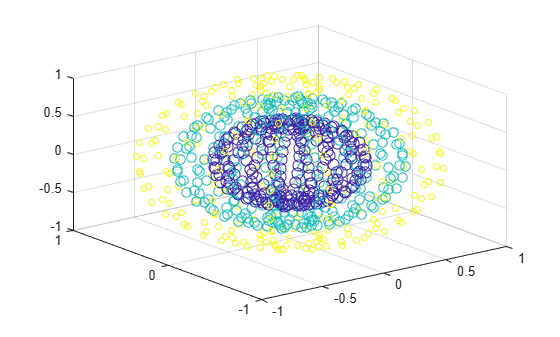Use an RGB triplet color value to set the marker face color. Starting in R2014b, you can use dot notation to set properties. If you are using an earlier release, use the `set` function instead.

`h.MarkerFaceColor = [0 0.5 0.5];`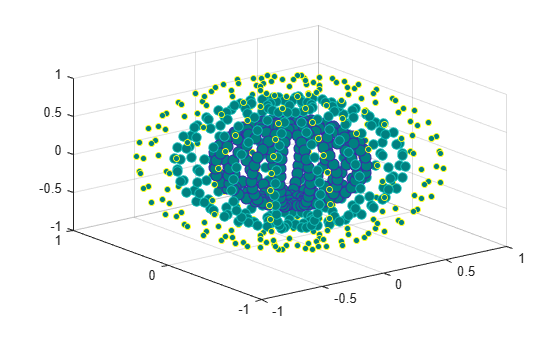## Input Arguments

collapse all

x values, specified as a vector. `X`, `Y`, and `Z` must be vectors of equal length.

Data Types: `single` | `double` | `int8` | `int16` | `int32` | `int64` | `uint8` | `uint16` | `uint32` | `uint64` | `categorical` | `datetime` | `duration`

y values, specified as a vector. `X`, `Y`, and `Z` must be vectors of equal length.

Data Types: `single` | `double` | `int8` | `int16` | `int32` | `int64` | `uint8` | `uint16` | `uint32` | `uint64` | `categorical` | `datetime` | `duration`

z values, specified as a vector. `X`, `Y`, and `Z` must be vectors of equal length.

Data Types: `single` | `double` | `int8` | `int16` | `int32` | `int64` | `uint8` | `uint16` | `uint32` | `uint64` | `categorical` | `datetime` | `duration`

Marker area, specified as a scalar, a vector, or `[]`. The values in `S` must be positive. The units for area are points squared.

• If `S` is a scalar, then `scatter3` plots all markers with the specified area.

• If `S` is a row or column vector, then each entry in `S` specifies the area for the corresponding marker. The length of `S` must equal the length of `X`, `Y` and `Z`. Corresponding entries in `X`, `Y`, `Z` and `S` determine the location and area of each marker.

• If `S` is empty, then the default size of 36 points squared is used.

Example: `50`

Example: `[36,25,25,17,46]`

Marker color, specified as a an RGB triplet, a three-column matrix of RGB triplet, a vector, or one of the color options in the table.

An RGB triplet is a three-element row vector whose elements specify the intensities of the red, green, and blue components of the color. The intensities must be in the range `[0,1]`; for example, `[0.4 0.6 0.7]`. Alternatively, you can specify some common colors by name. This table lists the long and short color name options and the equivalent RGB triplet values.

OptionDescriptionEquivalent RGB Triplet
`'red'` or `'r'`Red`[1 0 0]`
`'green'` or `'g'`Green`[0 1 0]`
`'blue'` or `'b'`Blue`[0 0 1]`
`'yellow'` or `'y'`Yellow`[1 1 0]`
`'magenta'` or `'m'`Magenta`[1 0 1]`
`'cyan'` or `'c'`Cyan`[0 1 1]`
`'white'` or `'w'`White`[1 1 1]`
`'black'` or `'k'`Black`[0 0 0]`

If you have three points in the scatter plot and want the colors to be indices into the colormap, specify `C` as a three-element column vector.

Example: `'y'`

Example: `[1,2,3,4]`

Marker, specified as one of the markers in this table.

ValueDescription
`'o'`Circle
`'+'`Plus sign
`'*'`Asterisk
`'.'`Point
`'x'`Cross
`'square'` or `'s'`Square
`'diamond'` or `'d'`Diamond
`'^'`Upward-pointing triangle
`'v'`Downward-pointing triangle
`'>'`Right-pointing triangle
`'<'`Left-pointing triangle
`'pentagram'` or `'p'`Five-pointed star (pentagram)
`'hexagram'` or `'h'`Six-pointed star (hexagram)
`'none'`No markers

Axes object. If you do not specify an axes, then `scatter3` plots into the current axes.

### Name-Value Pair Arguments

Specify optional comma-separated pairs of `Name,Value` arguments. `Name` is the argument name and `Value` is the corresponding value. `Name` must appear inside quotes. You can specify several name and value pair arguments in any order as `Name1,Value1,...,NameN,ValueN`.

Example: `'MarkerFaceColor','red'` sets the marker face color to red.

The properties listed here are only a subset. For a complete list, see Scatter Properties.

Width of marker edge, specified as a positive value in point units.

Example: `0.75`

Marker outline color, specified `'flat'`, an RGB triplet, a hexadecimal color code, a color name, or a short name. The default value of `'flat'` uses colors from the `CData` property.

For a custom color, specify an RGB triplet or a hexadecimal color code.

• An RGB triplet is a three-element row vector whose elements specify the intensities of the red, green, and blue components of the color. The intensities must be in the range `[0,1]`; for example, ```[0.4 0.6 0.7]```.

• A hexadecimal color code is a character vector or a string scalar that starts with a hash symbol (`#`) followed by three or six hexadecimal digits, which can range from `0` to `F`. The values are not case sensitive. Thus, the color codes `'#FF8800'`, `'#ff8800'`, `'#F80'`, and `'#f80'` are equivalent.

Alternatively, you can specify some common colors by name. This table lists the named color options, the equivalent RGB triplets, and hexadecimal color codes.

Color NameShort NameRGB TripletHexadecimal Color CodeAppearance
`'red'``'r'``[1 0 0]``'#FF0000'``'green'``'g'``[0 1 0]``'#00FF00'``'blue'``'b'``[0 0 1]``'#0000FF'``'cyan'` `'c'``[0 1 1]``'#00FFFF'``'magenta'``'m'``[1 0 1]``'#FF00FF'``'yellow'``'y'``[1 1 0]``'#FFFF00'``'black'``'k'``[0 0 0]``'#000000'``'white'``'w'``[1 1 1]``'#FFFFFF'``'none'`Not applicableNot applicableNot applicableNo color

Here are the RGB triplets and hexadecimal color codes for the default colors MATLAB® uses in many types of plots.

RGB TripletHexadecimal Color CodeAppearance
`[0 0.4470 0.7410]``'#0072BD'``[0.8500 0.3250 0.0980]``'#D95319'``[0.9290 0.6940 0.1250]``'#EDB120'``[0.4940 0.1840 0.5560]``'#7E2F8E'``[0.4660 0.6740 0.1880]``'#77AC30'``[0.3010 0.7450 0.9330]``'#4DBEEE'``[0.6350 0.0780 0.1840]``'#A2142F'`Example: `[0.5 0.5 0.5]`

Example: `'blue'`

Example: `'#D2F9A7'`

Marker fill color, specified as `'flat'`, `'auto'`, an RGB triplet, a hexadecimal color code, a color name, or a short name. The `'flat'` option uses the `CData` values. The `'auto'` option uses the same color as the `Color` property for the axes.

For a custom color, specify an RGB triplet or a hexadecimal color code.

• An RGB triplet is a three-element row vector whose elements specify the intensities of the red, green, and blue components of the color. The intensities must be in the range `[0,1]`; for example, ```[0.4 0.6 0.7]```.

• A hexadecimal color code is a character vector or a string scalar that starts with a hash symbol (`#`) followed by three or six hexadecimal digits, which can range from `0` to `F`. The values are not case sensitive. Thus, the color codes `'#FF8800'`, `'#ff8800'`, `'#F80'`, and `'#f80'` are equivalent.

Alternatively, you can specify some common colors by name. This table lists the named color options, the equivalent RGB triplets, and hexadecimal color codes.

Color NameShort NameRGB TripletHexadecimal Color CodeAppearance
`'red'``'r'``[1 0 0]``'#FF0000'``'green'``'g'``[0 1 0]``'#00FF00'``'blue'``'b'``[0 0 1]``'#0000FF'``'cyan'` `'c'``[0 1 1]``'#00FFFF'``'magenta'``'m'``[1 0 1]``'#FF00FF'``'yellow'``'y'``[1 1 0]``'#FFFF00'``'black'``'k'``[0 0 0]``'#000000'``'white'``'w'``[1 1 1]``'#FFFFFF'``'none'`Not applicableNot applicableNot applicableNo color

Here are the RGB triplets and hexadecimal color codes for the default colors MATLAB uses in many types of plots.

RGB TripletHexadecimal Color CodeAppearance
`[0 0.4470 0.7410]``'#0072BD'``[0.8500 0.3250 0.0980]``'#D95319'``[0.9290 0.6940 0.1250]``'#EDB120'``[0.4940 0.1840 0.5560]``'#7E2F8E'``[0.4660 0.6740 0.1880]``'#77AC30'``[0.3010 0.7450 0.9330]``'#4DBEEE'``[0.6350 0.0780 0.1840]``'#A2142F'`Example: `[0.3 0.2 0.1]`

Example: `'green'`

Example: `'#D2F9A7'`

## Output Arguments

collapse all

`Scatter` object. This is a unique identifier, which you can use to query and modify the properties of the `Scatter` object after it is created.